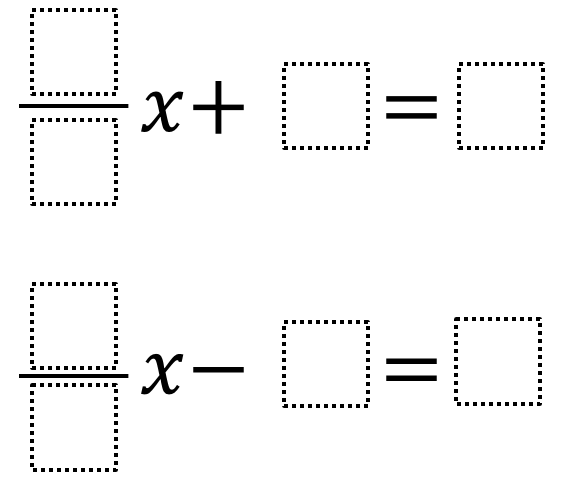Home > Grade 7 > Expressions & Equations > Exploring Equations

# Exploring Equations

Directions: For each problem below, use the digits 1 to 9 at most one time each, to fill in the boxes to find the the greatest value for x that you can.### Hint

Where should the 9 go or not go? Where should the 1 go or not go?

### Answer

(1/8)x + 2 = 9 yields a solution of 56.

(1/9)x – 8 = 7 and (1/9)x – 7 = 8 both have a solution of 135.

Source:  Chase Orton and Mark Goldstein

## Converting a Fraction to a Decimal

Directions: Using the digits 1 to 9, at most one time each, place a digit …